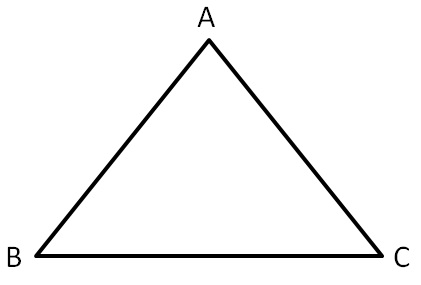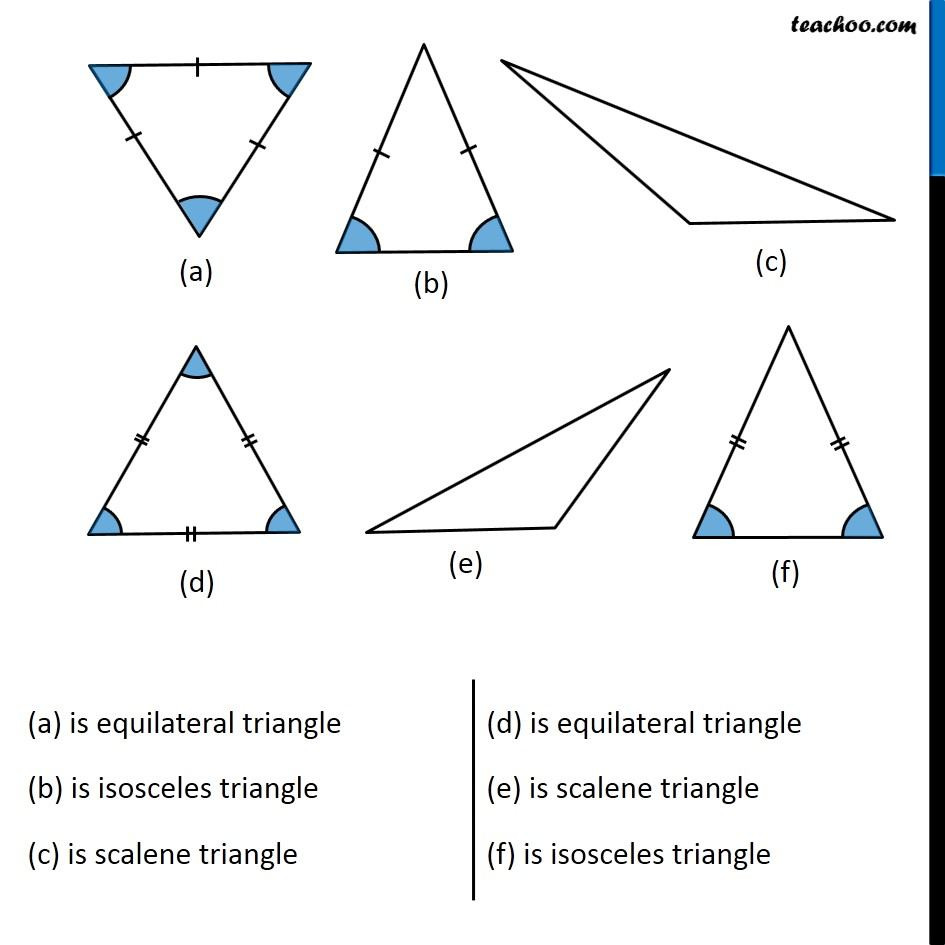Now, in ∆ABC,

there can be 3 cases

• All sides are different
• Two sides are same
• Three sides are same.

If all three sides are different, it is a scalene triangle.

If two sides are equal, it is an isosceles triangle.

If all three sides are same, it is an equilateral triangle.1. Chapter 6 Class 7 Triangle and its Properties
2. Concept wise
3. Basic Definitions

Transcript

(a) is equilateral triangle (b) is isosceles triangle (c) is scalene triangle (d) is equilateral triangle (e) is scalene triangle (f) is isosceles triangle

Basic Definitions